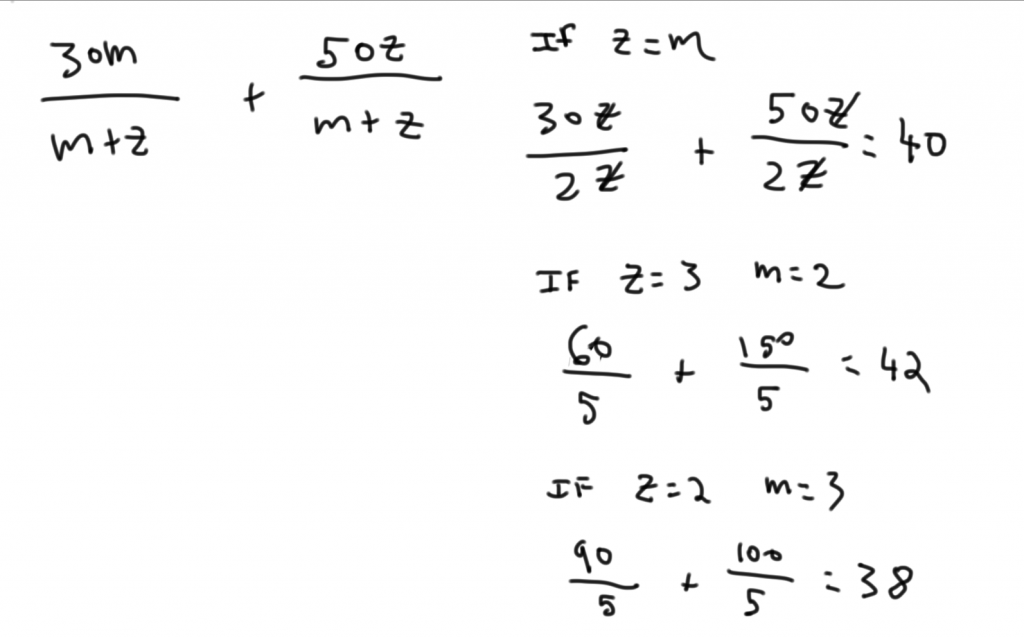Private GMAT Tutoring in NYC & Online

# GMAT Question of the Day - Data Sufficiency - Algebra/Threshold

In a certain company 2/5 of the employees are either engineers or scientists. What is the ratio of engineers to scientists?

(1) There are 75 employees in the company

(2) There are more than 3 times as many engineers as there are scientists

## GMAT Question of the Day Solution

It's good policy on GMAT Data Sufficiency to spend as much time as necessary to understand the question before moving on to the statements. The questions (and the information given in the question) are your friends. In this question of the day there isn't a ton of information in the question but still it might help to write out that 2/5's of the company is made up of Engineers and Scientists and that we are looking for E/S.

Statement 1 tells us that that E + S = 30. But we don't know what the mix is. Insufficient.

Statement 2 tells us that the ratio is greater than 3 to 1. So the ratio could be 3:1 or 4:1 or 7:2. Many possibilities. Insufficient.

Putting the statements together provides some limitations but not enough of them to narrow the ratio down. We could have 29 engineers and 1 scientist or 28 engineers and 2 scientists. Both ratios are greater than 3 to 1. Multiple possibilities. Insufficient.

# GMAT Question of the Day - Data Sufficiency - Inequalities

If m and z are positive numbers is 30m/(m+z) + 50z/(m+z) greater than 39?

(1) z/m > 1

(2) m/(m+z) < 1/2

## GMAT Question of the Day Solution:

Look at this as a weighted average question. If m = z then the answer will end up in the middle of 30 and 50. In this case 40. So the real question here is: z > m?  If this is confusing then I would suggest trying some numbers to make this concept very clear. Make  z=m, z > m, and finally m > z. Hopefully that will shed some light on how this question works (I worked this out at the bottom).

(1) Simplify the algebra to z > m. If z is greater than m then the value of the expression must be closer to 50 than 30. So the expression must be greater than 39. Sufficient.

(2) If the first part of the expression has less than half of the weight than the total expression must be closer to 50 then 30. So the expression must be greater than 39. Sufficient.# CONTACT

Atlantic GMAT

405 East 51st St.

NY, NY 10022

(347) 669-3545

info@AtlanticGMAT.com